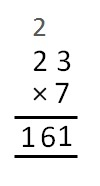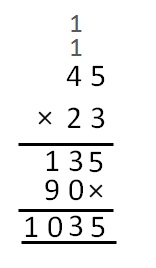Multiplication of Decimals

Chapter 2 Class 7 Fractions and Decimals
Concept wise

## Multiplication of Decimals

While multiplying decimals

• We remove decimal point, and convert into fractions
• We try to make denominator as 10, 100, 1000, … and solve
• And in the end, we convert fraction into decimals|

Let’s take some examples

2.83 × 4

= 2.83/100 × 4

= (283 × 4)/100

= 1132/100

= 11.322.3 × 4.5

= 23/10 × 45/10

= (23 × 45)/100

= (45 × 23)/100

= 1035/100

= 10.356.7 × 100

= 67/10 × 100

= 67 × 10

= 670.

0.008 × 10

= 0008/1000 × 10

= 8/1000 × 10

= 8/100

= 008/100

= 0.08

0.2 × 0.4

= 02/10 × 04/10

= 2/10 × 4/10

= (2 × 4)/(10 × 10)

= 8/100

= 008/100

= 0.08

### Transcript

Multiplication of Decimals While multiplying decimals We remove decimal point, and convert into fractions We try to make denominator as 10, 100, 1000, … and solve And in the end, we convert fraction into decimals Let’s take some examples 2.83 × 4 = 2.83/100 × 4 = (283 × 4)/100 = 1132/100 = 11.32 2.3 × 4.5 = 23/10 × 45/10 = (23 × 45)/100 = (45 × 23)/100 = 1035/100 = 10.35 6.7 × 100 = 67/10 × 100 = 67 × 100 = 670 0.008 × 10 = 0008/1000 × 10 = 8/1000 × 10 = 8/100 = 008/100 = 0.08 0.2 × 0.4 = 02/10 × 04/10 = 2/10 × 4/10 = (2 × 4)/(10 × 10) = 8/100 = 008/100 = 0.08